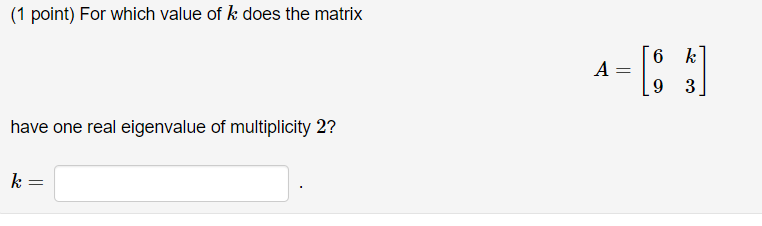Home / Expert Answers / Algebra / 1-point-for-which-value-of-k-does-the-matrix-a-69k3-have-one-real-eigenvalue-of-multiplic-pa822

# (Solved): (1 point) For which value of k does the matrix A=[69k3] have one real eigenvalue of multiplic ...(1 point) For which value of does the matrix have one real eigenvalue of multiplicity 2 ?

We have an Answer from Expert